# 二、加载数据集

2. colab运行时间有时限，长时间不操作（大概20分钟左右）会导致当前训练的数据被回收
3. 猫狗大战数据集是没有标签的，需要自己定义Dataset类加载数据

（1）colab上传和解压大数据集

• 解压drive上的cat_dog.rar文件，命令为

! apt-get install rar
!unrar x "/content/drive/Colab/人工智能课/cat_dog.rar" "/content/drive/Colab/人工智能课/"


解压过程如下：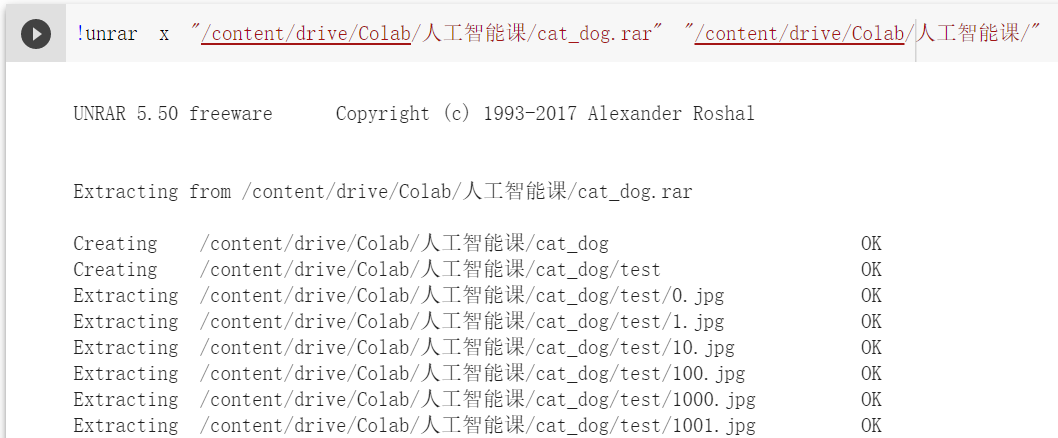!cp -i /content/drive/Colab/人工智能课/cat_dog.rar /content/

• 在虚拟机上解压压缩文件：

! apt-get install rar
! unrar x cat_dog.rar


运行过程如下：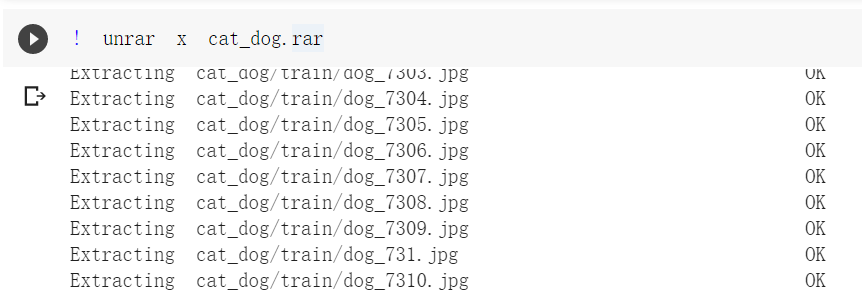（2）阻止Colab自动掉线

function ClickConnect(){
console.log("Working");
document
.querySelector("#top-toolbar > colab-connect-button")
.querySelector("#connect")
.click()
}

setInterval(ClickConnect,60000)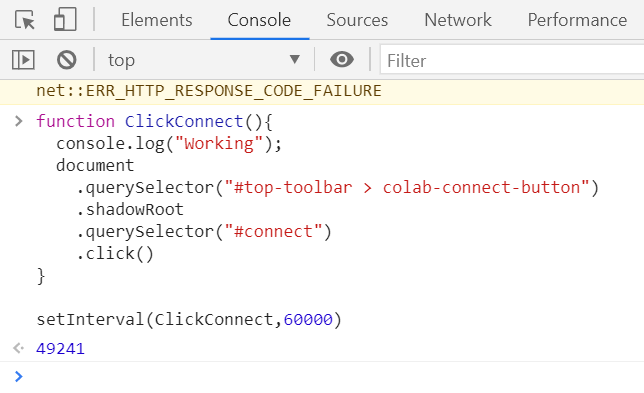（3）猫狗大战数据集是没有标签的，需要自己定义Dataset类才能加载数据

# 三、数据预处理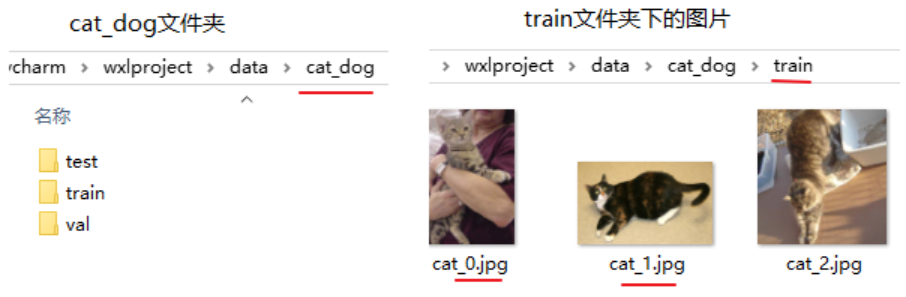from torch.utils.data import Dataset,DataLoader
# 创建自己的类：MyDataset,继承 Dataset 类
class MyDataset(Dataset):
super(MyDataset, self).__init__()
file_path = data_path + txt
file = open(file_path, 'r', encoding='utf8')
imgs = []
for line in file:
line = line.split()
imgs.append((line,line.rstrip('\n')))

self.imgs = imgs
self.transform = transform
self.target_transform = target_transform
self.data_path = data_path

# 可以通过索引进行条用，如data
def __getitem__(self, index):
# 按照索引读取每个元素的具体内容
imgName, label = self.imgs[index]
# imgPath = self.data_path + imgName
imgPath = imgName
# 调用那张图片读哪张，最大限度发挥GPU显存
if self.transform is not None:
img = self.transform(img)
label = torch.from_numpy(np.array(int(label)))
return img, label

def __len__(self):
# 数据集的图片数量
return len(self.imgs)

# 定义读取文件的各式
return Image.open(path).convert('RGB')


（1）首先，读取cat_dog文件夹下的图片路径

#读一个文件夹下的所有文件名称
filename = []
for root, dirs, files in os.walk(file_dir):
filename = files #当前路径下所有非目录子文件
break #这里只要图片文件，执行一次即可退出
return filename


（2）然后将文件名格式化为竞赛要求的类型，这里cat标签为0，dog为1

# 将文件名格式化为要求的类型，这里cat标签为0，dog为1
def format_inputAndlabel(file_dir):
format_result = []
for n in filename:#cat为0，dog为1
if "cat" in n:
format_result.append(n+" 0")
else:
format_result.append(n+" 1")
return format_result


（3）分别传入train、test、val路径读取数据

# 格式化读取train、test、val
format_train_result = format_inputAndlabel("cat_dog/train")
format_test_result = format_inputAndlabel("cat_dog/test")
format_val_result = format_inputAndlabel("cat_dog/val")


（4）由于自定义的DataSet必须知道文件路径，所以先将格式化的文件名写入文件里，再用自定义的MyDataset读取

def convert_format(content):
result = []
for t in content:
v = t.split('.')
result.append(int(v))
return result
# 写入train、val文件
def write_file(path,file_prefix,content):
with open(path, 'w', encoding='utf8') as f:
for line in content:
f.write(file_prefix+line+'\n')
# 写入test文件，由于读取时候文件名是乱序的，因此要先排序
def write_test_file(path,test_file_prefix,content):
content=convert_format(content)
content.sort() #排序
with open(path, 'w', encoding='utf8') as f:
for line in content:
f.write(test_file_prefix+str(line)+'.jpg 0'+'\n')# test文件没有标签，默认用0填充就行

# 因为自定义的DataSet必须知道文件路径，所以先将格式化的文件名写入文件里，再用自定义MyDataset读取
write_file(path="cat_dog/train.txt",file_prefix="cat_dog/train/",content=format_train_result)
write_file(path="cat_dog/val.txt",file_prefix="cat_dog/val/",content=format_val_result)
write_test_file(path="cat_dog/test.txt",test_file_prefix="cat_dog/test/",content=format_test_result)


（5）对数据进行预处理变换

from torch.utils.data import Dataset,DataLoader
import torchvision.transforms as transforms
# 预处理设置
normalize = transforms.Normalize(mean=[0.485, 0.456, 0.406], std=[0.229, 0.224, 0.225])
train_transformer = transforms.Compose([
transforms.Resize(256),
transforms.transforms.RandomResizedCrop((224), scale = (0.5,1.0)),
transforms.RandomHorizontalFlip(),
transforms.ToTensor(),
normalize])

# val和test是类似的，训练的时候可以多一些增强，这里只做验证就可以
val_transformer = transforms.Compose([
transforms.Resize(224),
transforms.CenterCrop(224),
transforms.ToTensor(),
normalize
])


（6）使用MyDataset加载图片路径文件

# 数据集加载方式设置
cmd_path='cat_dog/'
trainset = MyDataset(txt='train.txt',data_path=cmd_path,transform=train_transformer)
valset = MyDataset(txt='val.txt',data_path=cmd_path,transform=val_transformer)
testset = MyDataset(txt='test.txt',data_path=cmd_path,transform=val_transformer)
print('训练集：',trainset.__len__())
print('验证集：',valset.__len__())
print('测试集：',testset.__len__())
"""

"""


（7）使用torch.utils.data.DataLoader加载图片数据，并将其放入dataloaders_dict

batchsize=128
train_loader = DataLoader(trainset, batch_size = batchsize, drop_last = False, shuffle = True)
val_loader = DataLoader(valset, batch_size = batchsize, drop_last = False, shuffle = False)
test_loader = DataLoader(testset, batch_size = batchsize, drop_last = False, shuffle = False)


# 四、构建VGG模型

VGG 模型如下图所示，主体由三种元素组成：

• 卷积层（CONV）是发现图像中局部的 pattern
• 全连接层（FC）是在全局上建立特征的关联
• 池化（Pool）是给图像降维以提高特征的 invariance(不变性)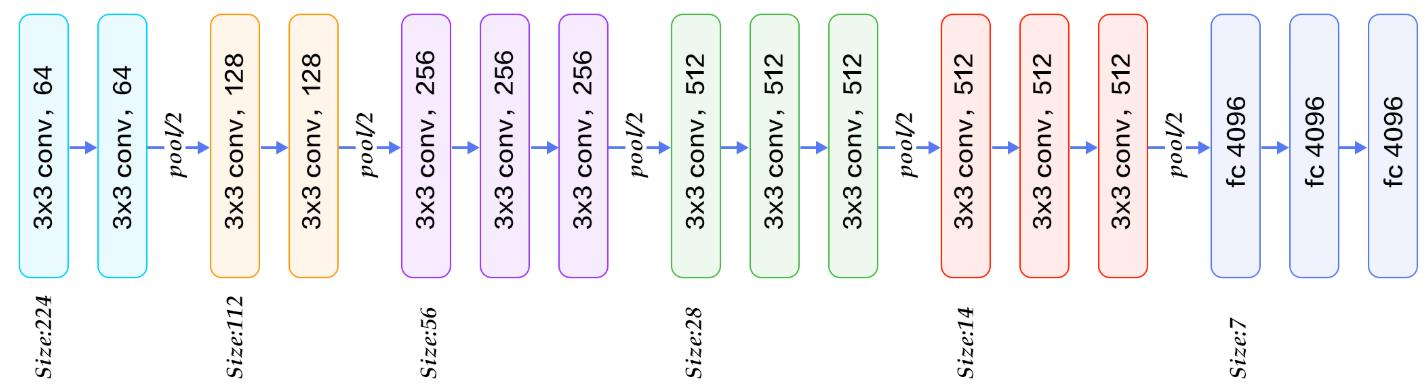def set_parameter_requires_grad(model, feature_extracting):
if feature_extracting:
for param in model.parameters():


def initialize_model(num_classes, feature_extract, use_pretrained=True):
# 初始化模型变量
model_vgg = None
# 加载预训练模型
model_vgg = models.vgg16(pretrained=use_pretrained)
# 更改输出层
model_vgg.classifier = nn.Linear(4096, num_classes)
return model_vgg

model_vgg_new = initialize_model(num_classes=2,feature_extract = True,use_pretrained=True)
print(model_vgg_new.classifier)


Sequential(
(0): Linear(in_features=25088, out_features=4096, bias=True)
(1): ReLU(inplace=True)
(2): Dropout(p=0.5, inplace=False)
(3): Linear(in_features=4096, out_features=4096, bias=True)
(4): ReLU(inplace=True)
(5): Dropout(p=0.5, inplace=False)
(6): Linear(in_features=4096, out_features=2, bias=True)
(7): LogSoftmax(dim=1)
)


# 五、训练VGG模型

（1）创建损失函数和优化器

'''

'''
# 损失函数
criterion = nn.NLLLoss()
# 学习率
lr = 0.001
# 优化器


（2）判断是否存在GPU设备，并将model切换到相应的device

device = torch.device("cuda:0" if torch.cuda.is_available() else "cpu")
print('Using gpu: %s ' % torch.cuda.is_available())
model_vgg_new.to(device)


（3）训练模型

'''

'''
def train_model(model, dataloaders, criterion, optimizer, num_epochs=25):
since = time.time()
val_acc_history = []

best_model_wts = copy.deepcopy(model.state_dict())
best_acc = 0.0

for epoch in range(num_epochs):
print('Epoch {}/{}'.format(epoch, num_epochs - 1))
print('-' * 10)

# 每个epoch都进行训练和验证
for phase in ['train', 'val']:
if phase == 'train':
model.train()  # 将模型设置为训练模式
else:
model.eval()   # 将模型设置为验证模式

running_loss = 0.0 # 记录训练时的loss下降过程
running_corrects = 0

# 遍历数据
inputs = inputs.to(device)
labels = labels.to(device)
# 梯度初始化
# 前向传播
outputs = model(inputs)
loss = criterion(outputs, labels.long())
# 得到预测结果
_, preds = torch.max(outputs, 1)
# 仅在训练时更新梯度，反向传播，backward + optimize
if phase == 'train':
loss.backward()
optimizer.step()
# statistics
running_loss += loss.item() * inputs.size(0)
running_corrects += torch.sum(preds == labels.data)

print('{} Loss: {:.4f} Acc: {:.4f}'.format(phase, epoch_loss, epoch_acc))

# 将验证集上结果最好的一次训练存储下来
if phase == 'val' and epoch_acc > best_acc:
best_acc = epoch_acc
best_model_wts = copy.deepcopy(model.state_dict())
if phase == 'val':
val_acc_history.append(epoch_acc)

print()

time_elapsed = time.time() - since
print('Training complete in {:.0f}m {:.0f}s'.format(time_elapsed // 60, time_elapsed % 60))
print('Best val Acc: {:4f}'.format(best_acc))

return model, val_acc_history

# 训练
model_new_vgg, hist = train_model(model_vgg_new, dataloaders_dict, criterion, optimizer_vgg, num_epochs=4)



import matplotlib.pyplot as plt
%matplotlib inline
import numpy as np
plt.title(u"val acc plot")
plt.xlabel(u"epoch")
plt.ylabel(u"val acc")
acc= hist
plt.xticks(range(len(acc)))
plt.plot(acc)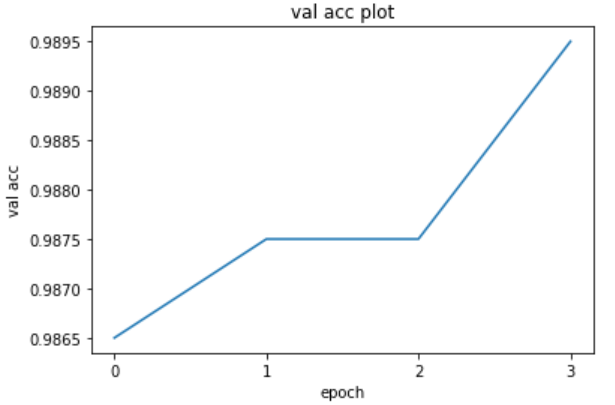# 六、保存与测试模型

（1）保存训练好的模型

pytorch保存和加载模型有两种方式，不同的保存方式对应不同的读取方式，两者各有利弊。

torch.save(model_new_vgg, 'model_new_vgg.pt')


model = initialize_model(num_classes=2,feature_extract = True,use_pretrained=True)
model.to(device)


（2）对模型进行测试

def test_model(model, test_loader):
model.eval() #把训练好的参数冻结
total,correct = 0,0
pos = 0
inputs = inputs.to(device)
outputs = model(inputs)
_, preds = torch.max(outputs, 1)
pred_outputs[pos:pos+len(preds)]=preds.cpu().numpy()
pos += len(preds)
return pred_outputs



（3）将测试结果写入cat_dog_result.csv

with open("cat_dog_result.csv", 'w') as f:
f.write("{},{}\n".format(i, pred_outputs[i]))


!cp -i /content/cat_dog_result.csv /content/drive/


（4）提交测试结果

cat_dog_result.csv提交到AI研习社猫狗大战–经典图像分类题，现在就让我们见证奇迹的时刻吧！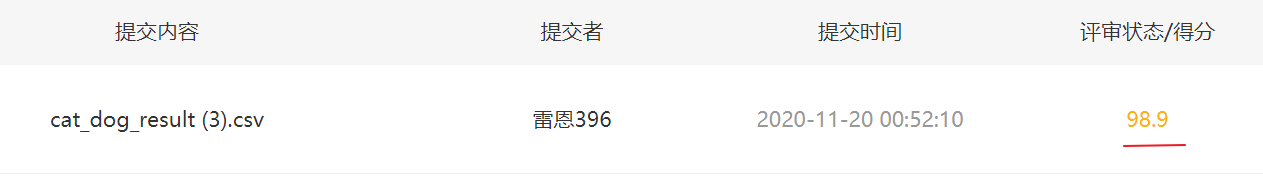# 七、总结

03-111万+

### “相关推荐”对你有帮助么？

•非常没帮助
•没帮助
•一般
•有帮助
•非常有帮助被折叠的  条评论 为什么被折叠?到【灌水乐园】发言¥2 ¥4 ¥6 ¥10 ¥20余额支付 (余额：-- )扫码支付获取中扫码支付点击重新获取扫码支付1.余额是钱包充值的虚拟货币，按照1:1的比例进行支付金额的抵扣。
2.余额无法直接购买下载，可以购买VIP、C币套餐、付费专栏及课程。余额充值